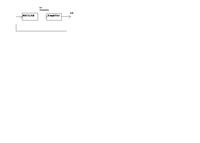# Tutorial on ADS -MATLAb Cosimulation

Status
Not open for further replies.

#### Manjularamkumar

##### Member level 1hi,

I need any tutorial regarding ADS-Matlab cosimulation.

I have to implement a optimization algorithm for amplifier. I couldnt write the equations for the amplifier .It is very difficult.

So I have chosen Cosimulation. Using the output of ADS , the matlab function could generate input variables for amplifier.

I have a doubt. 1. Please see this figure.Can I able to connect like this figure? I read many threads regarding ADS o/p which is stored in ASCII txt format and call in Matlab using function "import". I also give it like this. whether the loop (amplifier to matlab )connected is correct?

2. Please give any example or tutorial or thesis for simple example.

Status
Not open for further replies.